# Introduction to Neural Networks (Psy 5038): Python & MCMC¶

### MCMC with PyMC¶

PyMC 2.3.4 is the production version.

For introductions to PyMC and Probabilistic programming in Python see: https://www.youtube.com/watch?v=XbxIo7ScVzc and https://www.youtube.com/watch?v=WESld11iNcQ

PyMC has been rewritten as PyMC3. See: https://pymc-devs.github.io/pymc3/index.html and https://github.com/pymc-devs/pymc3

For various examples of code for Bayesian methods, see https://github.com/aloctavodia/Doing_bayesian_data_analysis for Python examples from John K. Kruschke's book (1rst ed.), "Doing Bayesian Data Analysis" translated from R to Python.

And scikit-learn, http://scikit-learn.org/stable/, is a general resource for machine learning, including probablistic methods.

In [ ]:



### Neural network motivation¶

In the next lectures, we will begin to look at large-scale system neural architectures for vision. The basic structure consists of lateral, feedforward, and feedback computations. A conjectured function of lateral processing is to link features of a similar type, for example similar orientations from nearby spatial positions. Texture synthesis Gibbs sampler is an example of a lateral linking process which links similar colors. These can be extended to other more abstract features. And later, we will look at methods for linking features at a similar level of abstraction.

Information can also be linked based on a model of "common cause". Often there is more than one explanation for any piece of data, and one needs to estimate the probability of where it came from. These kinds of models are naturally hierarchical. One example is segmentation where one decides which pixels belong to one object (e.g. in the foreground) vs. another (the background). Segmentation or grouping can be based on linking features of similar type, and/or based on distinguishing common causes.

To illustrate a basic computation, here we look a simple toy version of the problem in which a scalar data point could come from one of two processes. We don't know which process led to any observed value, we don't know the central tendencies or variances of the processes. But we assume we have enough data to make good guesses.

Specifically, we'll see how a gaussian mixture model can be modeled using MCMC sampling with PyMC. The model is simple enough that we could implement an MCMC solution without the help of PyMC. However, using PyMC will help to see how to use it to model more complex problems.

Later we'll see how from a machine learning perspective, grouping operations can also be modeled as a form of unsupervised learning, based on similarity measures, and be studied in the context of clustering algorithms such as EM and K-means. In a later lecture, we'll look at the EM solution and apply it to a clustering problem, applied to distinguishing different possible occluding surfaces.

This example is based on and uses code from Chapter 3 of Probabilistic Programming and Bayesian Methods for Hackers

In :
import numpy as np
import pymc as pm
import matplotlib.pyplot as plt
import matplotlib as mlp
import pandas as pd

%matplotlib inline

#We'll use this next function to show the graphical relations
from IPython.display import Image

/Users/kersten/anaconda/envs/pymc2/lib/python2.7/site-packages/matplotlib/font_manager.py:273: UserWarning: Matplotlib is building the font cache using fc-list. This may take a moment.
warnings.warn('Matplotlib is building the font cache using fc-list. This may take a moment.')

In [ ]:


##### Synthetic data¶

There are a number of ways of drawing random numbers using Python packages. For example try np.random.normal(0,1). We'll use the scipy.stats module, because it supports a number of methods for many standard distributions.

Our true underlying model is a mixture of gaussians, with mixing parameter truep:

In [ ]:


In :
#?np.random.normal

In :
from scipy.stats import bernoulli,norm

truemean1=100.0
truemean2=200.0
truestd=25.0
truep=0.25

n=2000

data=np.zeros(n)
for i in xrange(len(data)):
p = bernoulli.rvs(truep)
data[i]=p*norm.rvs(truemean1, truestd)+(1-p)*norm.rvs(truemean2, truestd)

plt.hist(data);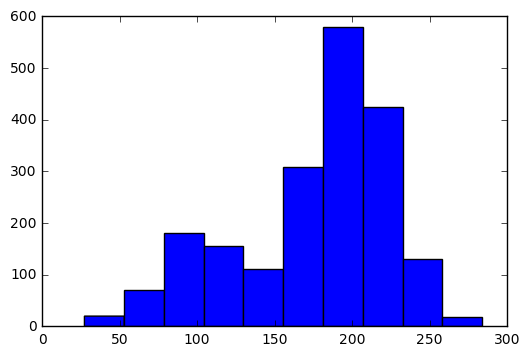In [ ]:



### Generative model¶

Here's where we begin to use PyMC. Suppose we were presented with the data, but don't know the generative process. So we begin to guess the possible general structure.

We will assume that each data point comes from one of two normal distributions, but we don't know the probability p that it comes from the first. The true value is truep (above), which we would like to estimate. Let's define a PyMC random variable p that reflects our uncertainty. The true value of p is fixed for the process that generated the data and since we don't know its value, assume it is uniformly distributed between 0 and 1. We will want to estimate the value of p.

Now imagine that for each data point we'd also like to estimate whether it came from the first or second distribution. We define a second random (vector) variable "assignment" that depends on p. In the language of graphical models, 'p' is the parent of the 'assignment' (vector) variable. The assignment vector thus contains n random values, one for each data point.

In :
p = pm.Uniform("p", 0, 1)

assignment = pm.Categorical("assignment", [p, 1 - p], size=np.shape(data))


These variables are called stochastic variables.

Experiment with:

?pm.Uniform
pm.Uniform("test", 0, 1,value=.2,observed=True).value
pm.Uniform("test", 0, 1,observed=False).value

p.value

?pm.Categorical
assignment.value[:10]
In :
assignment.value[:10]

Out:
array([1, 0, 0, 0, 1, 0, 1, 0, 1, 1])

We also want to estimate the two means of the normal distributions assumed to have generated the data.

We'll assume the priors on the means of the two generating distributions are normally distributed. We just guess that their centers are 110 and 210, and allow for our uncertainty with a guess that each of their standard deviations is 10. You could explore how much bad guesses cost in terms of iterations to convergence (or in general, non-convergence).

Call these random variables 'centers'.

Note that these standard deviations reflect our uncertainty in the means, not the variability in data generated by either of the two assumed distributions. We also want to estimate standard deviations for each of the two distributions.

PyMC works with precision $\tau$, rather than standard deviation $\sigma$:

$$\tau = {1 \over \sigma^2}$$

Call the precisions 'taus'. And we'll assume a uniform distribution of the standard deviations from 0 to 100.

In :
centers = pm.Normal("centers", [110, 210], [10**-2, 10**-2], size=2)
taus = 1.0 / pm.Uniform("stds", 0, 100, size=2) ** 2

###### Likelihood¶

We've defined the priors. Now we need to describe how the generating variables could produce the observed data. PyMC has a "decorator" function pm.deterministic, that is used to model functions in which the inputs, if known, deterministically specify the outputs. IF we did know which distribution a data point came from, we could assign it to the appropriate mean (center 0 or 1) with certainty. Similarity for the taus.

The functions below map an assignment of 0 or 1, to a set of parameters representing the centers and taus.

In :
@pm.deterministic
def center_i(assignment=assignment, centers=centers):
return centers[assignment]

@pm.deterministic
def tau_i(assignment=assignment, taus=taus):
return taus[assignment]

# OK. Here's the final summary line representing our model that relates the centers and taus to the data
# We assume that observations, the data, can be explained by the mixture model

observations = pm.Normal("obs", center_i, tau_i, value=data, observed=True)


Try:

print "Random assignments: ", assignment.value[:4], "..."

print "Assigned center mean: ", center_i.value[:4], "..."

print "Assigned precision: ", tau_i.value[:4], "..."

print centers[assignment].value



These show current values, which may be accidents of the initial set-up, or reflect requests for random draws. Ideally, their exact values shouldn't matter to the final estimates.

While center_i and tau_i are deterministic functions, their values while running the simulation will vary reflecting the stochastic property of their parents. So they are still random variables.

In [ ]:



Finally we ask PyMC to create a model class.

In :
# create a model class
model = pm.Model([p, assignment, observations, taus, centers])


The model will get handed off to pm.MCMC(model) class below to run the simulations. But first, let's get a graphical view of what we've done using the directed graph function: pm.graph.dag.

## Graphical view¶

Ellipses represent nodes to be estimated or are fixed. Triangles represent deterministic functions. It looks more complicated than it is.

In :
pm.graph.dag(pm.MCMC(model)).write_png('dag.png')
Image('dag.png')

Out: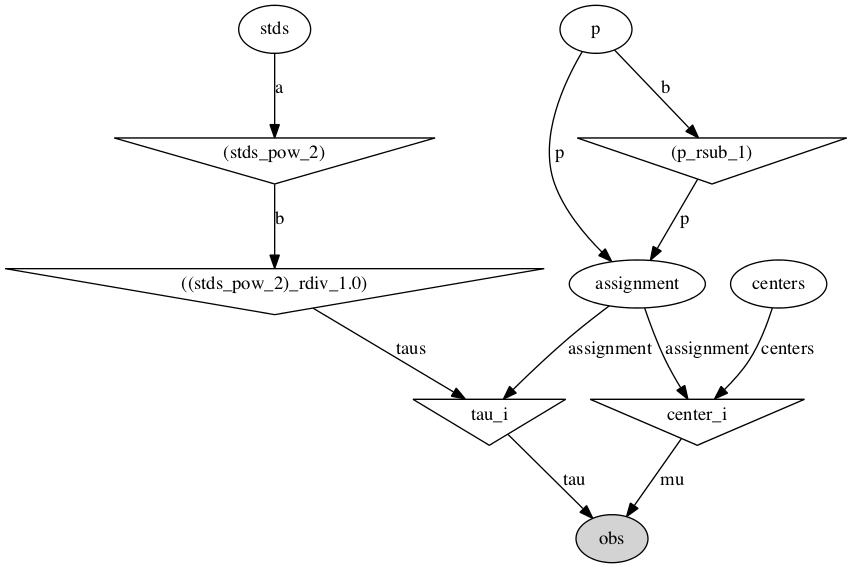In [ ]:



## MCMC simulation¶

It can help to try to estimate the MAP value of the parameters. This can then be used as the starting condition for MCMC. We'll leave this step out for now.

In :
#map1 = pm.MAP(model)
#map1.fit() #stores the fitted variables' values in foo.value


Try 500 samples

In :
mcmc = pm.MCMC(model)
#now use MCMC's sample method
mcmc.sample(500)

 [-----------------100%-----------------] 500 of 500 complete in 0.3 sec

## Results¶

Let's plot up the traces, which are the marginals over the posterior probability of each of the estimates of the means (center0, center 1), their standard deviations (), and the probability of assignment.

Figure code from "Probabilistic Programming and Bayesian Methods for Hackers", ( (http://nbviewer.ipython.org/github/CamDavidsonPilon/Probabilistic-Programming-and-Bayesian-Methods-for-Hackers/blob/master/Chapter3_MCMC/IntroMCMC.ipynb)

In :
from IPython.core.pylabtools import figsize

figsize(12.5, 9)
plt.subplot(311)
lw = 1
center_trace = mcmc.trace("centers")[:]

# for pretty colors.
colors = ["#348ABD", "#A60628"] \
if center_trace[-1, 0] > center_trace[-1, 1] \
else ["#A60628", "#348ABD"]

plt.plot(center_trace[:, 0], label="trace of center 0", c=colors, lw=lw)
plt.plot(center_trace[:, 1], label="trace of center 1", c=colors, lw=lw)
plt.title("Traces of unknown parameters")
leg = plt.legend(loc="upper right")
leg.get_frame().set_alpha(0.7)

plt.subplot(312)
std_trace = mcmc.trace("stds")[:]
plt.plot(std_trace[:, 0], label="trace of standard deviation of cluster 0",
c=colors, lw=lw)
plt.plot(std_trace[:, 1], label="trace of standard deviation of cluster 1",
c=colors, lw=lw)
plt.legend(loc="upper left")

plt.subplot(313)
p_trace = mcmc.trace("p")[:]
plt.plot(p_trace, label="$p$: frequency of assignment to cluster 0",
color="#467821", lw=lw)
plt.xlabel("Steps")
plt.ylim(0, 1)
plt.legend()

Out:
<matplotlib.legend.Legend at 0x112e2d110>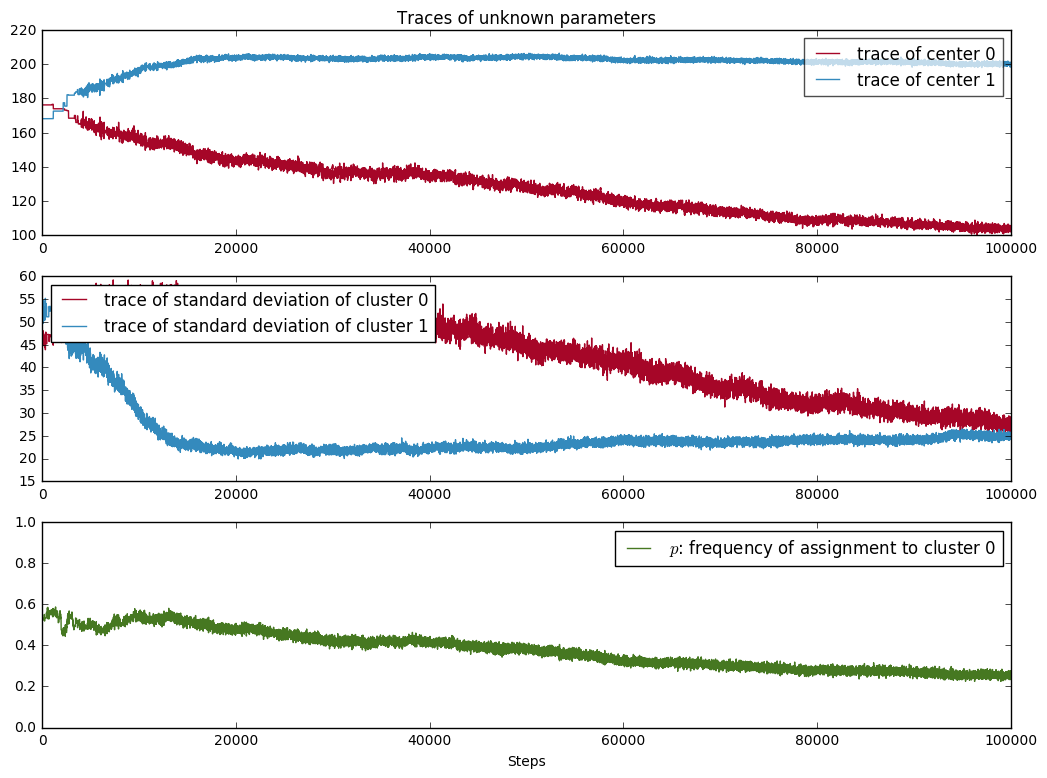The traces don't appear to have converged yet. mcmc remembers its samples, so we can continue where we left off and gather an additional 100,000.

In :
mcmc.sample(100000)

 [-----------------100%-----------------] 100000 of 100000 complete in 60.3 sec

Let's get the means of the traces and compare with the true values

In :
print mcmc.trace('centers')[:,0].mean(),mcmc.trace('centers')[:,1].mean(),mcmc.trace('p')[:].mean()

print truemean1, truemean2, truep

128.95125323 200.588231264 0.379026198557
100.0 200.0 0.25


But we shouldn't include samples from the early part of the chain when estimating the means.

Try estimating the means using only the last 50000 steps of the traces.

If there is insufficent storage, one can specify a burn-in period where the first part of the chain gets discarded. Further, there may be concern over the independence of samples. One can also just keep every mth sample. This is called thinning, and can be specified during the sampling call.

In :
print mcmc.trace('centers')[50000:100000:5,0].mean(),mcmc.trace('centers')[50000:100000:5,1].mean(),mcmc.trace('p')[50000:100000:5].mean()

print truemean1, truemean2, truep

113.078986385 201.893448214 0.298833417335
100.0 200.0 0.25

In :
?pm.MCMC.sample


One can check for corrrelations by looking the auto-correlations of the traces. Plotting the histogram gives some sense of whether how the space is being sampled.

In :
from pymc.Matplot import plot as mcplot

mcplot(mcmc.trace("centers", 2), common_scale=False)

Plotting centers_0
Plotting centers_1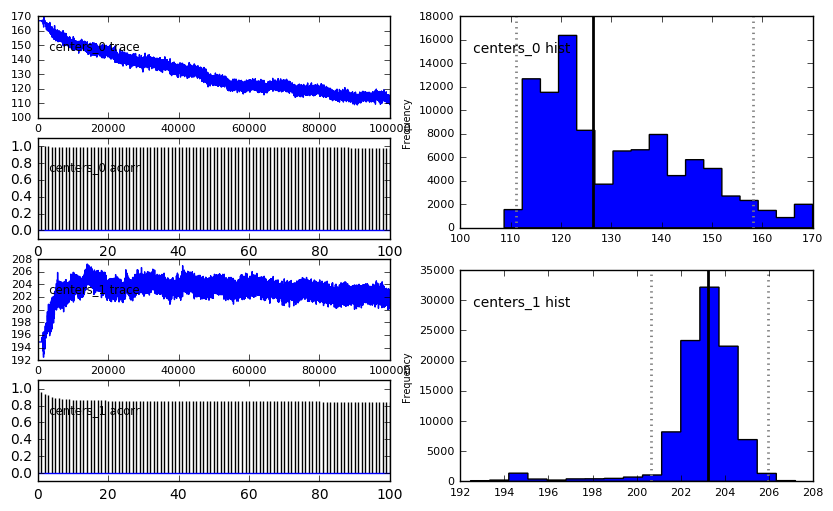In :
pm.Matplot.plot(p)

Plotting p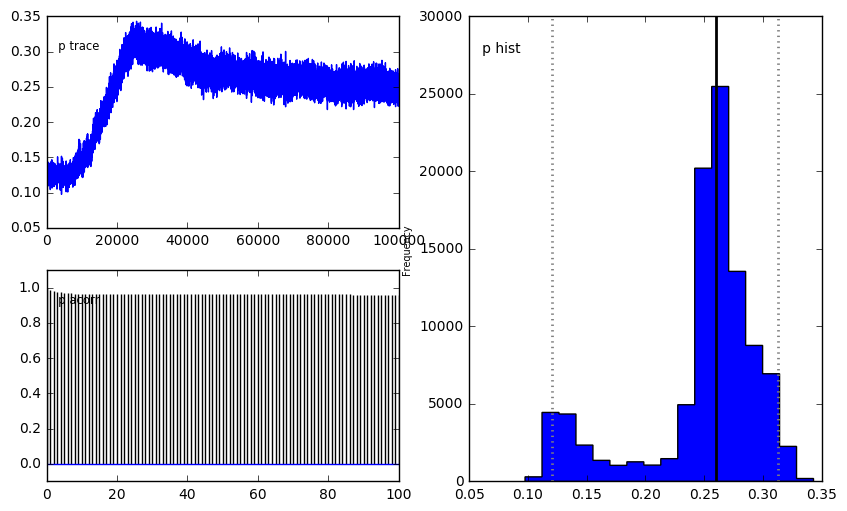Once we leave toy problems, questions of convergence, adequate mixing become more of a challenge. A primary motivation for the writing of PyMC3 was to employ more recent advancements in sampling methods, and which scale up to higher dimensional spaces.

See Chapter 3 from Probabilistic Programming and Bayesian Methods for Hackers ( (http://camdavidsonpilon.github.io/Probabilistic-Programming-and-Bayesian-Methods-for-Hackers/) for a discussion in the context of the above example.

# Further information and references on probabilistic programming using python and other software tools¶

HDDM: Hierarchical Bayesian estimation of the Drift-Diffusion Model in Python. http://www.ncbi.nlm.nih.gov/pmc/articles/PMC3731670/

Non-python, probablistic computation for cognition using Church: https://probmods.org/

In [ ]:
from IPython.display import YouTubeVideo

In :
YouTubeVideo("WESld11iNcQ")

Out:

For an introduction to PyMC3 see:

In :
YouTubeVideo("VVbJ4jEoOfU")

Out:

For an overview of MCMC software, its history and applications see the talk by pymc's original author, Chris Fonnesbeck.

In :
YouTubeVideo("XbxIo7ScVzc")

Out:

For detailed background on sampling using Python, see:

In :
YouTubeVideo("vOBB_ycQ0RA")

Out:
In [ ]: